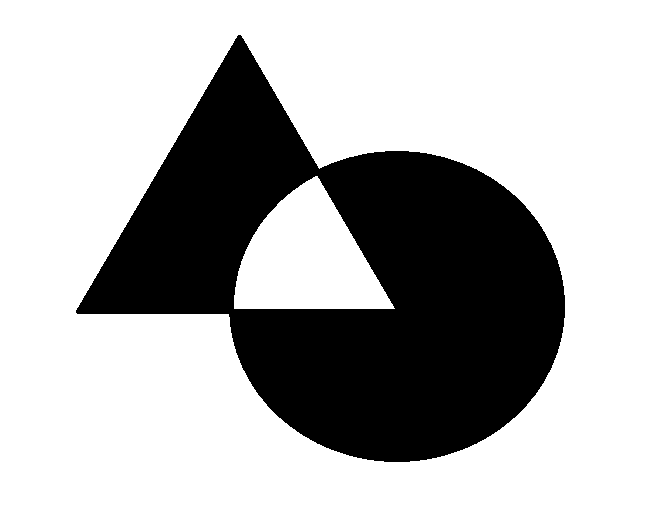# Center of Mass - Part 2Consider a thin uniform circular disk of radius $r$. A thin uniform equilateral triangle of side length $2r$ is then added and it has a vertex at the center of the circle and it has the same density as the circular disk. The triangle and the circle overlap partially and exist in the same plane. This area of overlap between the triangle and the circle is then cut out leaving part of the original disk and the additional part of the triangle. The center of mass of this resulting shape is a distance $d$ from the center of mass of the original uniform disk. This distance $d$ can be expressed in the form $\frac{a}{b\pi + 3\sqrt{3}}r$ where $a$ and $b$ are integers and $r$ is the radius of the uniform circular disk. What is the value of $a + b$?

×